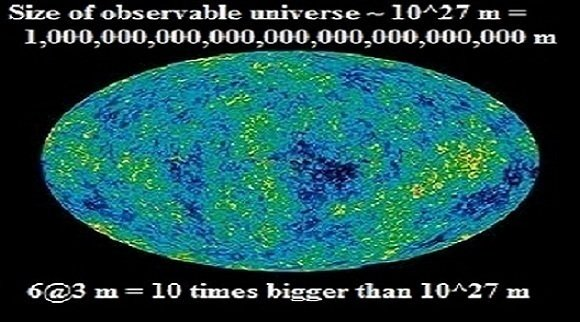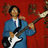# WORLD LEADERS IN COSMOLOGY: CHANGE THE WAY TO EXPRESS DISTANCE IN UNIVERSE

Petition Closed# WORLD LEADERS IN COSMOLOGY: CHANGE THE WAY TO EXPRESS DISTANCE IN UNIVERSETHOMAS NGUYEN started this petition to INTERNATIONAL ASTRONOMICAL UNION World Leaders in Cosmology and

To avoid using of extremely big numbers to express distances in Universe. Solution: Using a new mathematics operation called ReExpOp (math symbol is @)

Example:  5@3 = (5^5)^5 = 2.9802322388e+17 = 298,023,223,880,000,000

2@5 = (((2^2)^2)^2)^2 = 65,536

3@4 = ((3^3)^3)^3 = 7,625,597,484,987

6@3 = (6^6)^6 ~ 10^28 = 10x10^27

6@3 m = 10x10^27 m = 10 times bigger than Size of observable universe

(Answers can be referred from ReExpOp Table, like Time Table chart for multiplication operation)